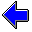Java Security Settings:
This web page employs Java, which requires specific security settings for correct operation.
If the applets on this page do not run correctly, consult the Virtual Chemistry Experiments FAQ
or the Physlet Physics web site for establishing the correct security settings.

# Gas Laws

## Boyle's Law

### Concepts

Boyle's Experiment

Some of the earliest quantitative measurements were performed on gases. One early study was conducted by Robert Boyle in 1662.

Robert Boyle employed a J-shaped piece of glass tubing that was sealed on one end. A gas (air) was trapped in the sealed end of the tube and varying amounts of mercury were added to the J-shaped tube to vary the pressure of the system. Boyle systematically varied the pressure and measured the volume of the gas. These measurements were performed using a fixed amount of gas and a constant temperature. In this way Boyle was able to examine the pressure-volume relationship without complications from other factors such as changes in temperature or amount of gas.

The manometer that was employed in the previous experiment can also be used to perform Boyle's experiment. A small amount of air is trapped in the sealed end of the manometer. The scale that is used to read the height of a column of mercury can also be used to read the height of the column of air. (You will need to estimate the position of the top of the tube, which is curved.) The inside diameter of the tube, 4.286 cm, and the height of the column of air can be used to calculated the volume of air.

The pressure of the gas will be varied in exactly the same manner as Robert Boyle varied the pressure: mercury will be added or removed from the open end of the manometer. After changing the pressure (by changing the amount of mercury in the manometer), the pressure and volume are recorded.

Data Analysis

Once the volume-pressure data has been obtained, the next challenge is to determine the mathematical relationship between the two properties. Although an enormous number of relationships are possible, one likely possibility is that the volume will be directly related to the pressure raised to some power:

V = CBL P a

The exponent a is expected to be independent of the mass of gas and temperature; the goal is to determine the value of a from the "experimental" data. The constant CBL is expected to vary with the mass of gas and the temperature; at this point, this constant is not of interest.

A simple way to determine the value of a is to prepare a plot of ln V vs ln P. If the proposed relationship is valid (and it might not be valid), this plot should yield a straight line of slope a. Thus the linearity of the plot serves as a test of our original hypothesis (that the volume-pressure relation may be described by the equation shown above).

### Experiment

Objective
• Determine how the volume of a gas changes with the pressure for a fixed amount of gas and temperature.
Part 1
 The basic details of the experiment have been described above. The open tube of the manometer is exposed to the atmosphere. Consequently the atmospheric pressure (Patm) must be added to the pressure exerted by the column of mercury. The first step in the experiment is thus to measure the atmospheric pressure using the manometer containing no trapped air.

Part 2

A sample of air is now trapped in the closed end of the manometer.

Carefully measure the heights of the columns of mercury and the column of trapped air. (The trapped air has artificially been given a light green color.) Use this data to calculate the volume of the trapped gas and the pressure. The inside diameter of the manometer tube is 4.286 cm. You will need to use the value for Patm measured in Part 1 of this experiment.

Change the amount of mercury in the manometer and once again measure the volume and pressure. Continue this process until data is obtained for at least five different pressures.

Notice that sometimes the column of mercury on the left is higher than that on the right and sometimes the reverse is true. Why does this occur? Make sure you take this effect into account in calculating the pressure.

For each pair of volume-pressure values, enter the data in the table. The point will automatically be plotted on the graph.

Carefully examine the plots of V vs P and ln V vs ln P and determine the value of a.

Pressure = torr
Volume = mL

Pressure
(torr)
Volume
(mL)

Plot Type
V vs P       ln V vs ln P

Slope =           Intercept =Pressure                     Calculations using Boyle's LawGas Laws Home PageVirtual Chemistry Home Page

BoylesLaw.html version 2.1